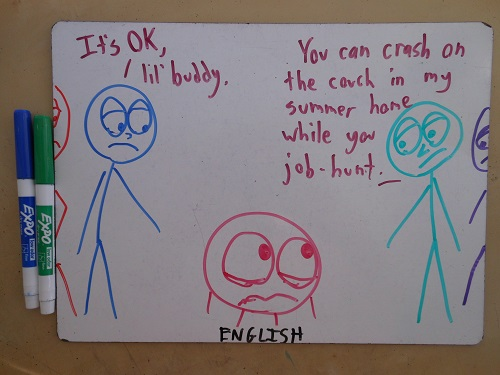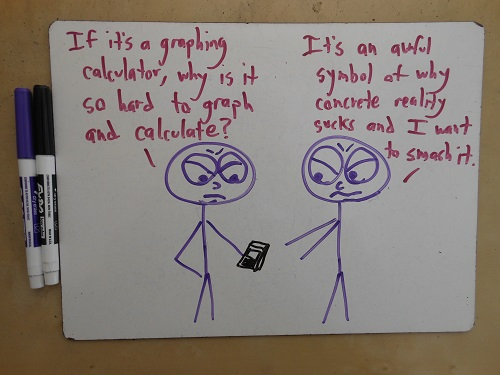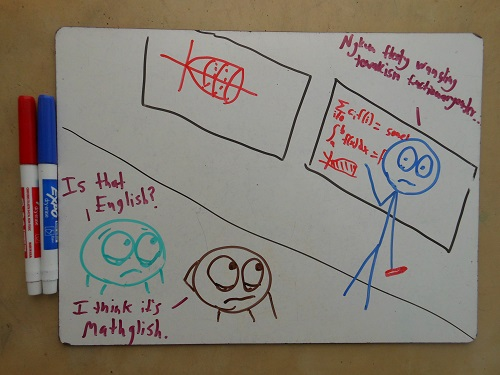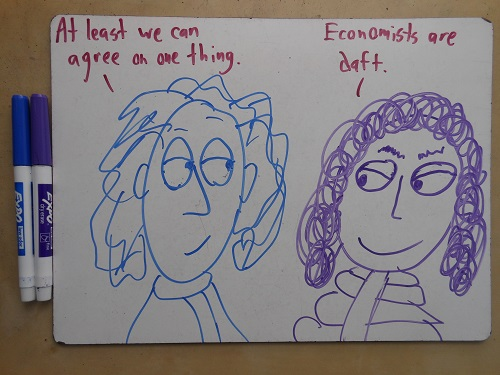# The Mathematical Dialect Quiz1. What do you call a rigorous demonstration that a statement is true?
1. If “proof,” then you’re a mathematician
2. If “experiment,” then you’re a physicist
3. If you have no word for this concept, then you’re an economist1. What do you call a slow, painful, computationally intense method of solving a problem?
1. If “engineering,” then you’re a mathematician
2. If “mathematics,” then you’re an engineer
1. What do you call a person who is in their first job after a PhD?
1. If “postdoc,” then you’re a mathematician or physicist
2. If “assistant professor,” then you’re an economist
3. If “wealthy,” then you’re a computer scientist
4. If you have no word for a job after a PhD, then you’re in the humanities, and you have our condolences1. What do you call a calculator with graphing capabilities?
1. If “an antique,” then you’re a computer scientist
2. If “my precious,” then you’re an engineer
3. If “the poor man’s Wolfram Alpha,” then you’re a mathematician
4. If “kinda hard to use,” then you’re an honest mathematician1. How do you pronounce “Pythagorean”?
1. If you pronounce it “pithAGorEan,” then you’re a mathematician
2. If you pronounce it “PITHaGORean,” then you’re a physicist
3. If you just mumble the word and hope no one notices, then you’re a TA1. What name do you use for the person who invented calculus?
1. If “Leibniz,” then you’re a mathematician
2. If “Newton,” then you’re a physicist
3. If “magical wizard,” then you’re probably not ready for grad school1. What do you say after successfully proving your point beyond all doubt?
1. If “QED,” then you’re a mathematician
2. If “the prosecution rests,” then you’re a mathematician with a flair for drama
3. If you do not believe proof beyond all doubt is possible, then you’re a scientist1. What do you call a simplified representation of reality, such as imagining a physical system with no friction or air resistance?
1. If “a model,” then you’re a computer scientist
2. If “an approximation,” then you’re an engineer
3. If you call this “reality,” then you’re an economist1. How do you refer to a piece of work that suffers from one small but visible mistake?
1. If “rough,” then you’re an engineer
2. If “as good as it’s going to get,” then you’re a computer scientist
3. If “worthless,” then you’re a mathematician1. What do you call a formal gathering of professionals from your field?
1. If “a conference,” then you’re a physicist
2. If “a start-up,” then you’re a computer scientist
3. If “an advisory panel to the president,” then you’re an economist
4. If “a game of D&D,” then you’re a mathematicianThanks for reading! If you prefer bad gifs to bad drawings, you might also check out The Math Aficionado’s Guide to High Fives.

## 67 thoughts on “The Mathematical Dialect Quiz”

1.Aliyaaaa says:

This is brilliant! Particularly from a Mathematics undergrad’s perspective!

2.fred hartshorn says:

I was an engineer with a Math degree…

3.Amcii C. Bellamy says:

Reblogged this on Subatomic-Visage: and commented:
Like a lot…thanks for post!

4.lablua says:

“1. What do you call a rigorous demonstration that a statement is true?”

D. If “steps to reproduce” or “reproduction”, then you’re a computer scientist.

5.gand3r says:

8. What do you call a simplified representation of reality, such as imagining a physical system with no friction or air resistance?

D. If “an abstraction,” then you’re a mathematician.

6.Hélène Wilkinson says:

Wonderful, thank you so much.

5. What’s a TA?

7. Wouldn’t Gödel have answered C? He was a mathematician…